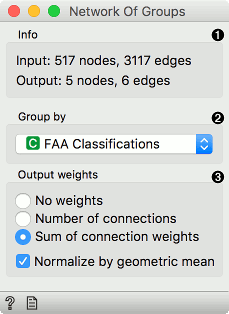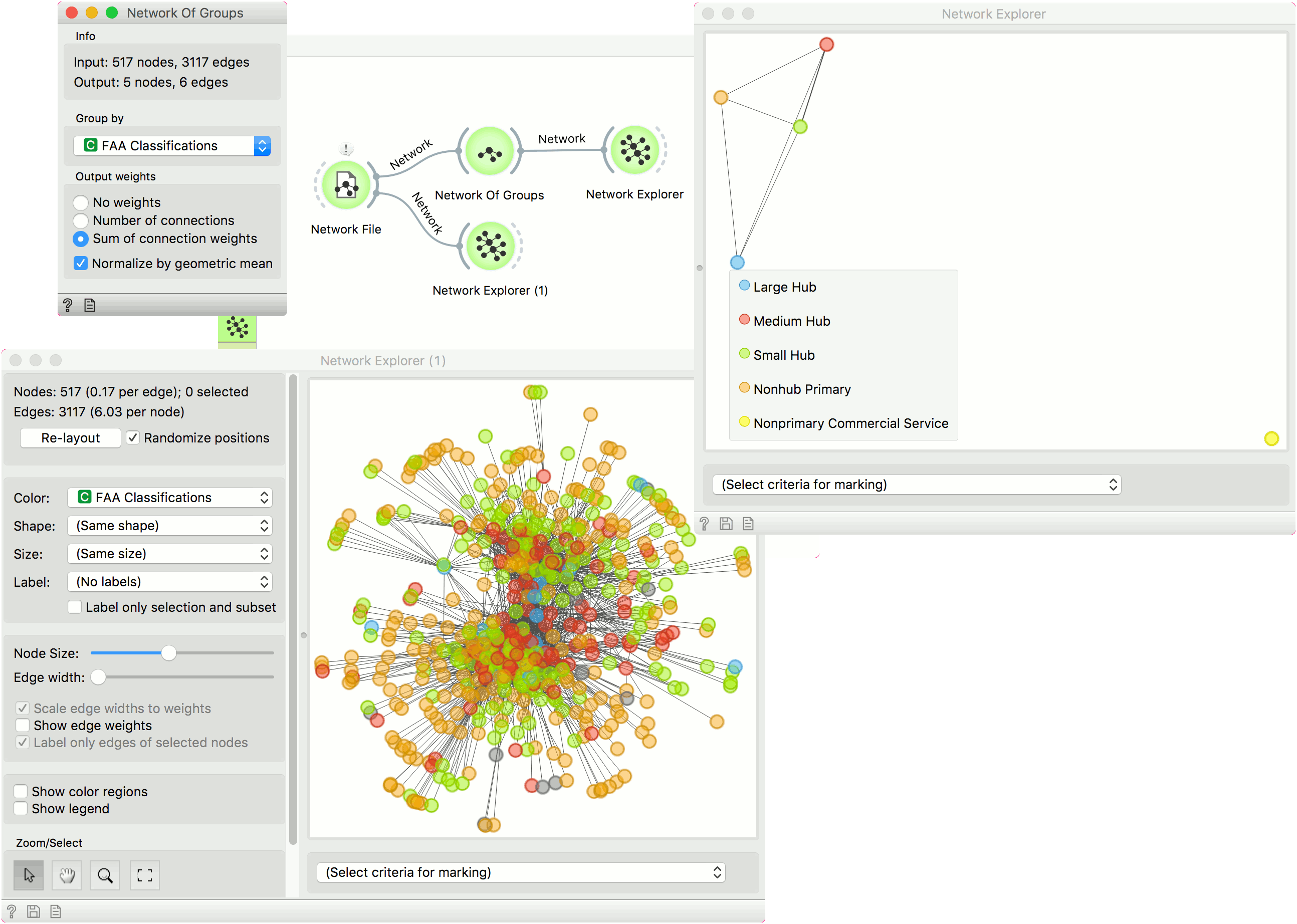# Network Of Groups

Group instances by feature and connect related groups.

Inputs

• Network: An instance of network graph.
• Data: Properties of a network graph.

Outputs:

• Network: A grouped network graph.
• Data: Properties of the group network graph.

Network of Groups is the network version of the group-by operation. Nodes with the same values of the attribute, selected in the dropdown, will be represented as a single node.1. Information on the input and output network.
2. Select the attribute to group by.
3. Compute weights:
• No weights: all weights are set to 1.
• Number of connections: weight edges by the number of connections between the groups.
• Sum of connection weights: weight edges by the sum of weights of connections between the groups. Normalize by geometric mean divides weights by the geometric mean of the number of connections between the two groups.

## Example

In this example we are using airtraffic data set, that we loaded in the Network File widget. We see the entire data set in Network Explorer (1).

Then we use Network of Groups to group the network by the FAA Classifications attribute. All nodes with the same value of this attribute will be represented as a single node in the output. There is an edge between the two nodes, if they share connections in the original network.

The grouped network is shown in Network Explorer.